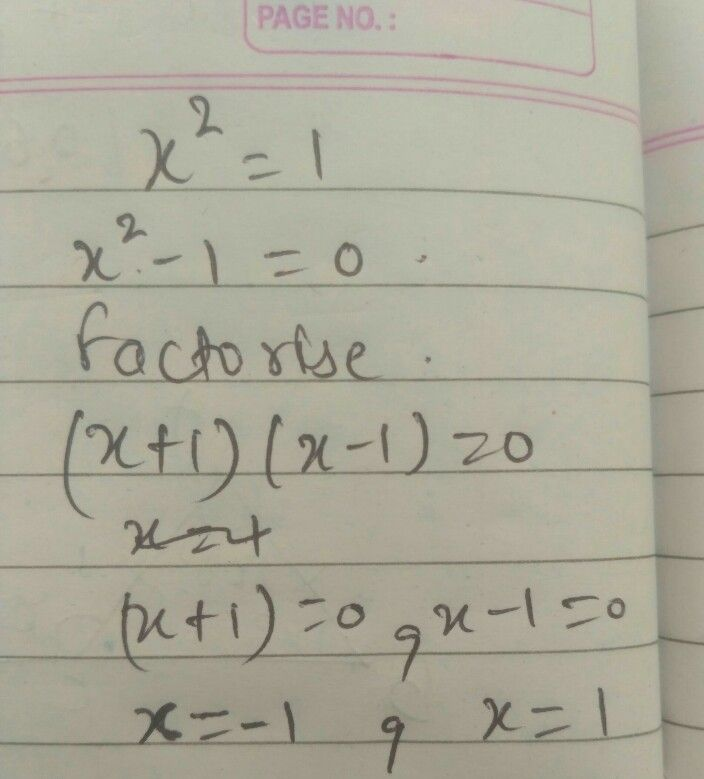Symbol
Problem$17$ What method can we use to solve a quadratic equation that can be written in the $\left(0xmx^{2}=12$ C. Extracting the Square Roots $A$ Quadratic Formula $1D$ Completing the Square $β.$ $1$ Factoring
7th-9th grade
Other
Search count: 106
SolutionQanda teacher - Reema03please evaluate my answer with 5 stars
thankyou
option B